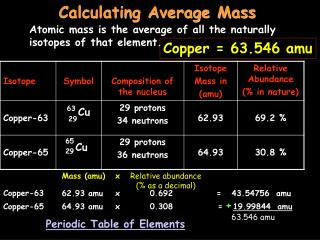DownloadDownload PresentationCalculating Average Mass

# Calculating Average Mass

Download Presentation## Calculating Average Mass

- - - - - - - - - - - - - - - - - - - - - - - - - - - E N D - - - - - - - - - - - - - - - - - - - - - - - - - - -
##### Presentation Transcript

1. Mass (amu) x Relative abundance (% as a decimal) Calculating Average Mass Atomic mass is the average of all the naturally isotopes of that element. Copper = 63.546 amu Cu Cu Copper-63 62.93 amu x 0.692 = 43.54756 amu + Copper-65 64.93 amu x 0.308 = 19.99844 amu 63.546 amu Periodic Table of Elements

2. C Carbon 6 Atomic # 12.011 Mass (amu) x Relative abundance (% as a decimal) Your Turn (Calculate Average Mass) 12 13 Carbon-12 12.000 amu x 0.9889 = 11.8668 amu + Carbon-13 13.003 amu x 0.0111 = 0.1443333 amu 12.0111333 amu 12.01 amu Atomic mass (average)

3. N Nitrogen 7 14.007 Mass (amu) x Relative abundance (% as a decimal) Your Turn (Calculate Average Mass) 14 7- 14 7 14-7=7 7 15 7- 15 7 15-7=8 7 35 17- 35 17 35-17=18 17 37 17- 37 17 37-17=20 17 Nitrogen-14 14.003 amu x 0.9963 = 13.9511889 amu + = 0.0555 amu Nitrogen-15 15.000 amu x 0.0037 14.0066889 amu 14.007 amu

4. Cl Chlorine 17 35.453 Mass (amu) x Relative abundance (% as a decimal) Your Turn (Calculate Average Mass) 14 7- 14 7 14-7=7 7 15 7- 15 7 15-7=8 7 35 17- 35 17 35-17=18 17 37 17- 37 17 37-17=20 17 Chlorine-35 34.969 amu x 0.7577 = 26.4960113 amu + = 8.9568618 amu Chlorine-37 36.966 amu x 0.2423 35.4528731 amu 35.453 amu

5. Pb Lead 82 207.20 Mass (amu) x Relative abundance (% as a decimal) Calculate Average Mass Mass # = p+ + n0 82p 122n 82p 124n 82p 125n 82p 126n 26.26% 20.82% 51.55% % abundance: 1.37% 204 207 Mass # 206 208 1.37 100 No amu mass shown. Use mass # instead. Lead-204 204 x 0.0137 = 2.7948 = 54.0956 Lead-206 206 x 0.2626 = 43.0974 Lead-207 207 x 0.2082 + = 107.224 Lead-208 208 x 0.5155 207.2118 207.21

6. He Helium 2 Atomic # 4.0026 Mass (amu) x Relative abundance (% as a decimal) Your Turn (Calculate Average Mass) 3 4 Helium-3 3.0160 amu x 0.000001 = 0.000003016 amu + Helium-4 4.0026 amu x 0.999999 = 4.002595997 amu 4.002599013 amu 4.0026 amu Atomic mass (average)